Next: Implementation Up: Moore theorem Previous: Moore theorem   Contents

Mathematical background

Let a system ofequations inunknowns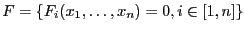each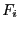being at least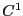. Let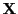be a range vector for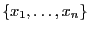,a point insideand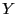an arbitrary nonsingular real matrix. Let define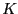as: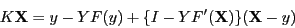and let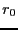be the norm of the matrix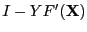. If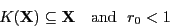then there is a unique solution  of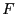in. This unique solution can be found using Krawczyk solving method (see section 2.10).

Jean-Pierre Merlet 2012-12-20